## Light Box

Author(s): Brandon Duran and Laurie Aiello
Demonstration Equipment - Teacher's Guide
SED 695B; Fall 2005

Principles illustrated:

• Reflection
• Refraction
• Snell's Law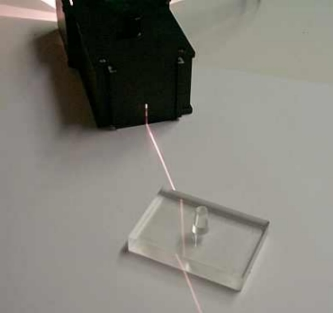Grade 7 Life Science:

6c: Students know light travels in straight lines if the medium it travels through does not change.
6d: Students know how simple lenses are used in a magnifying glass, the eye, a camera, a telescope, and a microscope.
6f: Students know light can be reflected, refracted, transmitted, and absorbed by matter.
6g: Students know the angle of reflection of a light beam is equal to the angle of incidence.

Physics:

4f: Students know how to identify the characteristic properties of waves: interference (beats), diffraction, refraction, Doppler effect, and polarization.

Materials
Explanation of principles involved

Included in Kit (see below for picture)

• Light box
• Bulb
• Light cover to get single or multiple lines of light.
• Acrylic Blocks of various shapes
• Colored Cardboard
• Colored Acetate
• Mirrors of various shapes

Needed Separately

Reflection on a plane mirror states that the angle of incidence is equal to the angle of reflection, as measured from the normal.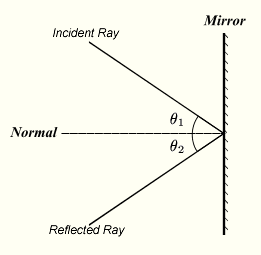Refraction is the bending of light as it travels through materials of different densities or indices of refraction. The amount of bending can be described using Snell's Law.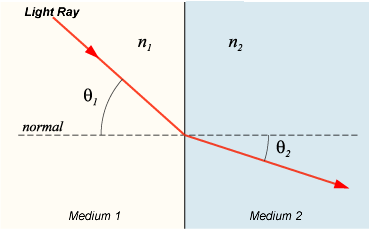Snell's law describes the relationship between the angles of incidence and refraction for a wave moving from one media to another with differing indices of refraction. The law is given by the formula: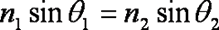Where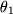is the angle of incidence (measured from the normal) and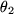is the angle of refraction (again measured from the normal) and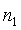and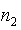are the indices of refraction for the initial and final media, respectively.

Procedures:

### Refraction with an extension to verify Snell's Law:

1. Set up the light box on a table so it only a single slit of light exits.
2. Place a sheet of paper on the table and tape it down.
3. On the paper, draw a line indicating the path of the light. Label this line "line 1"
4. Without moving the paper or the light box, place the acrylic block in the path of the light beam, such that the block is perpendicular to the entering line. You will hopefully see that the light has been refracted. Draw this new line, label it "line 2"
5. Turn the block slightly (as before, do not move the paper or the light box), and you should notice light bend more. Again, draw this new line and label it "line 3".
6. You may use lines 2 and 3 to verify Snell's law or to determine either index of refraction, given the other index of refraction. The index of refraction for air is 1, the index of refraction for acrylic is 1.3.
7. Play with other materials to determine their indices of refraction!

### Angle of Reflection vs Angle of Incidence

1. Place a plane mirror on a surface perpendicular to a table top.
2. Place a protractor flat against the mirror, in order to be able to measure the angle of incidence and the angle of reflection. It would be helpful to place a piece of paper under this with a Normal line drawn (recall that the Normal is at a 90 degree angle from the surface of the mirror).
3. In the light box, place the cover so that a single slit of light exits.
4. Aim the slit at the mirror where the line of normal touches the mirror at various angles. For each angle measure the angle of incidence and the angle of reflection, they should be the same!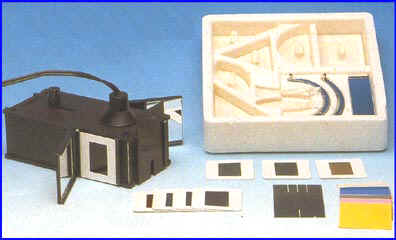A Picture of the supplied components, image from http://osawindia.net/Light_Box.jpg